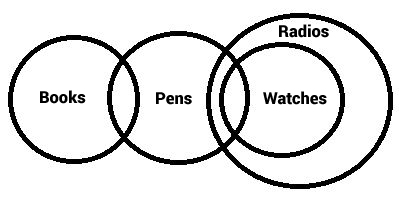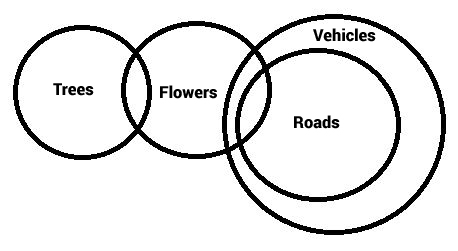# Syllogism Solved Questions and Answers for SBI Clerk Set-3

0
708

## Syllogism Solved Questions and Answers for SBI Clerk Set-3

Download SBI Clerk Syllogism Questions & Answers Set-3 PDF for SBI Clerk Prelims and Mains exam. Very Important SBI Clerk Syllogism Questions with solutions.

Instructions

In each of the question below are given three statements followed by two conclusion numbered I and II. You have to take the given statements to be true even if they seem to at variance from commonly known facts and then decide which of given conclusion logically follows from the statements disregarding commonly known facts Give answers–
A If only conclusion I follows
B If only conclusion II follows
C If either conclusion I or conclusion II follows
D If neither conclusion I nor conclusion II follows
E If both conclusion I and II follows

Question 1: statements:
All towns are cities.
All cities are urban.
Some urban are rural.
Conclusion:I. Some towns are rural.
II. All rural are towns.

a) If only conclusion I follows

b) If only conclusion II follows

c) If either conclusion I or conclusion II follows

d) If neither conclusion I nor conclusion II follows

e) If both conclusion I and II follows

Instructions

In each of the question below are given three statement followed by two conclusions numbered I and II. You have to take the given statements to

be true even if they seem to be at variance from commonly known facts and then decide which of the given conclusions logically follows from the statements

disregarding commonly known facts.

Question 2: Statements:All colleges are buildings.All buildings are concreteSome concrete are strongConclusions :I. Some colleges are strong.II. At least some strong are concrete.

a) If only conclusion I follows.

b) If only conclusion II follows.

c) If either conclusion I or conclusion II follows

d) If neither conclusion I nor conclusion II follows

e) If both conclusion I and II follow.

Instructions

In each of the following question below are given three statements followed by three conclusion numbered I, II, III. You have to take the given statements to be true even if they seem to be at variance from commonly known facts. Read all the conclusions and then decide which of the given conclusion logically follows from the given statements disregarding commonly known facts

Question 3: Statements:
Some towers are windows.
All windows are houses.
Some houses are temples.
Conclusions:
I: Some towers are temples.
II: some houses are towers.
III: Some temples are windows.

a) Only I follows

b) Only II follows

c) Only III follows

d) Only I and II follows

e) None of these

Instructions

In each question below are given two/three statements followed by two conclusion numbered I and II.You have to take the given statements to be true even if they seem to be at variance with commonly known fact.Read all the conclusions and then decide which of the given conclusion logically follows from the given statement disregarding commonly know facts.Give answer.

Question 4: Statements: All rings are circles.
All square are are rings.
No ellipse is a circle.
Conclusions:I. Some rings being ellipses is a possibility.
II. At least some circles are squares.

a) if only conclusion I follows.

b) if only conclusion II follows.

c) if either conclusion I or conclusion II follows.

d) if neither conclusion I nor conclusion II follows.

e) if both conclusion I and conclusion II follows.

Instructions

In each question below are two statements followed by two conclusions numbered I and II. You have to take the two given statements to be true even if they seem to be at variance from commonly known facts and then decide which of the given conclusions logically follows from the given statements disregarding commonly known facts.

Question 5: ‘’Statements’’ All gems are diamonds. All diamonds are rocks.
Conclusions: I. Atleast some rocks are gems.
II. All gems are rocks

a) only Conclusion I follows

b)  only Conclusion II follows

c) either Conclusion I or II follows

d)  neither Conclusion I nor II follows

e)  both Conclusions I and II follow

Instructions

In each questions below are two/three statements followed by two conclusions numbered I and II. You have to take the two/three given statements to be true even if they seem to be at variance from commonly known facts and then decide which one of the given conclusions logically follows from the given statements disregarding commonly known facts.

Question 6: Statements: All rings are circles. All squares are rings. No ellipse is a circle. Conclusions: I. Some rings being ellipses is a possibility
II. Atleast some circles are squares.

a) if only conclusion I follows

b) if only conclusion II follows

c) if either conclusion I or conclusion II follows

d) if neither conclusion I nor conclusion II follows

e) if both conclusion I and conclusion II follow

Instructions

In each of the question below are given four statements followed by four conclusions numbered I,II,III, and IV.You have to take the given statements to be true even if they seem to be at variance with commonly known facts Read all the conclusions and then decide which of the given conclusion logically follows from the given statements disregarding commonly known facts

Question 7: Statements:All birds are horses.
All horses are tigers
Some tigers are lions
Some lions are monkeys
Conclusion:I.Some tigers are horses
II.Some monkeys are birds
III.Some tigers are birds.
IV.Some monkeys are horses

a) Only I and III follows

b) Only I II and III follows

c) Only II,III and IV follows

d) All I, II, III and IV follows

e) None of these

Instructions

In each question below are four statements followed by four conclusions numbered I,II,III and IV You have to take the four given statements to be true even if they seem to be at variance with commonly known facts and then decide which of the given conclusions logically follows from the four given statements disregarding commonly known facts. Then decide which of the answer and indicate it on a),b),c),d) and e) is the correct answer and indicate it on the answersheet

Question 8: Statements:All stones are pearls.Some pearls are shells
Some shells are boxes. No box is container
Conclusions:I.Some stones are shells
II. No pearl is container
III.No shell is container
IV.Some pearls are containers

a) Only II follows

b) Only II & III follows

c) Only either II or IV follows

d) Only III follows

e) None of these

Instructions

In each question below are statements followed by two conclusions numbered I and II You have to take the two given statements to be true even if they seem to be at variance from commonly known facts and then decide which of the given conclusions logically follows from the given statements disregarding commonly known facts ?
(a)If only Conclusion I follows
(b)If only Conclusion II follows
(c)If either Conclusion I or II follows
(d)If neither Conclusion I nor II follows
(e)If both Conclusions I and II follow

Question 9: Statement All roads are streets No street is a highway
Conclusions

a) If only Conclusion I follows

b) If only Conclusion II follows

c) If either Conclusion I or II follows

d) If neither Conclusion I nor II follows

e) If both Conclusions I and II follow

Instructions

In each of the question below are given four statements followed by four conclusions numbered I, II III and IV You have to take the given statements to be true even if they seem to be at variance from commonly known facts Read all the conclusions and then decide which of the given conclusions logically follows from the given statement disregarding commonly known facts.

Question 10: Statements:      All papers are clips.
Some clips are boards
Some boards are lanes.         All lanes are roads
Conclusions:

II. Some lanes are clip

III. Some boards are papers     IV. Some roads are clips.

a) Only I and II follow

b) Only I and III follow

c) Only I II and III follow

d) Only II III and IV follow

e) None of these

Instructions

In each question below are given some statements followed by four conclusions numbered I, II, III and IV. You have to take the given statements to be true even if they seem at variance from commonly known facts. Read all the conclusions and then decide which of the given conclusions logically follows from the two given statements, disregarding commonly known facts.

Question 11: Statements: Some films are clouds
All rats are clouds
Some clouds are chairs
Conclusions: I. No film is chair
II. Some rats are films
III. Some clouds are rats
IV. Some chairs are rats

a) Only I and III follow

b) Either II or IV follows

c) No any conclusion follows

d) Only IV follows

e) None of these

Instructions

In each question below are two statements followed by two conclusions numbered I and II. You have to take the two given statements to be true even if they seem to be at variance from known facts and then decide which of the given conclusions logically commonly follows from the given statements disregarding commonly known facts.
Give answer a: if only conclusion I follows.
Give answer b: if only conclusion II follows.
Give answer c: if either conclusion I or II follows.
Give answer d: if both conclusions I and II follow.
Give answer e: if neither conclusion I nor II follows.

Question 12: Statements:
All buses are cars.
Some buses are trucks.
Conclusions:
I. Some buses are definitely not trucks.
II. At least some trucks are cars.

a) only conclusion I follows.

b) only conclusion II follows.

c) either conclusion I or II follows

d) both conclusion I and II follow.

e) neither conclusion I nor II follows.

Question 13: Statements:
Some gates are doors.
No gate is window.
Conclusions:
I. No door is window.
II. Some doors are definitely windows.

a) only conclusion I follows.

b) only conclusion II follows.

c) either conclusion I or II follows

d) both conclusion I nor II follow.

e) neither conclusion I nor II follows.

Instructions

In each question below are given three statements followed by four conclusions I II III and IV You have to take the given statements to be true even if they seem to be at variance with commonly known facts Read all the conclusions and then decide which of the given conclusion logically follow from given statements disregarding commonly Known facts

Question 14: Statements
Some books are pens.
Some pens are watches.
Conclusions
III.Some watches are books.
IV.Some books are watches.

a) All follow

b) Only I and III follow

c) Only II and IV follow

d) Only I and IV follow

e) None of these

Instructions

In each of the questions below are given three statements followed by four conclusions numbered I, II, III and IV. You have to take the given statements to be true even if they seem to be at variance from commonly known facts. Read all the conclusions and then decide which of the given conclusions logically follows from the given statements disregarding commonly known facts.

Question 15: Statements: Some trees are flowers. Some roads are flowers.
Conclusions:
I. Some vehicles are trees
II. Some vehicles are flowers

a) None follows

b) Only I and II follows

c) Only II follows

d) Only I, III and IV follow

e) All follow

The data can be intrepreted in Venn diagram from as: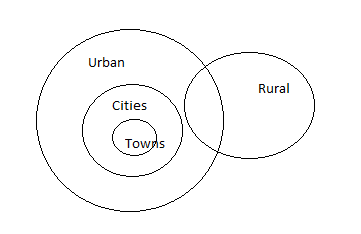Some towns are rural. We cannot say this with surety and this conclusion can be contradicted.
All rural are towns.This definitely cannot be concluded from Venn diagram.
Hence, both conclusion I and II do not follow.

The data can be intrepreted in Venn diagram form as: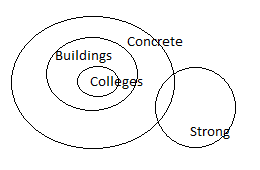Some colleges are strong.We cannot draw this conclusion with assurance.

At least some strong are concrete. This conclusion can be drawn with surety as apparent from Venn diagram.

Only II follows. Option B is correct.

The syllogism can be explained with following Venn Diagram.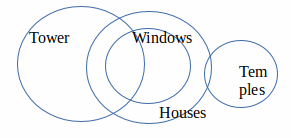Some towers are temples. This conclusion cannot be made.
Some houses are towers. This conclusion is true.
Some temples are windows. This conclusion again cannot be made with surety.

Only II follows.

ll rings are circles. Some circles are rings.
All square are are rings. Hence, some squares must be circles. Since no circle is an ellipse, no ring can be ellipse.
This can be seen from the following venn diagram.Only statement II follows.

The data can be intrepreted in Venn diagram form as: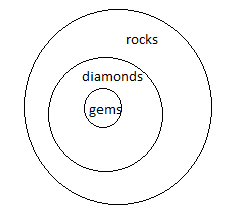Conclusions: I. Atleast some rocks are gems.This is true conclusion.
II. All gems are rocks. This is also a true comclusion as apparent from Venn diagram.
Both conclusion I and II follow.

The Venn diagram for the Syllogism is as follows: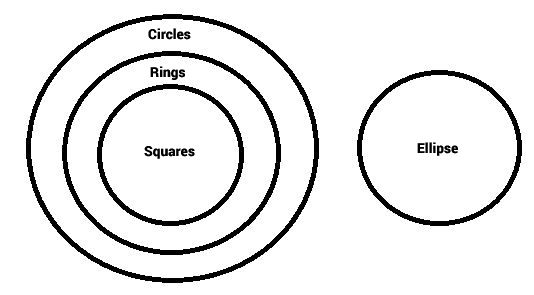Based on the diagram, some rings being ellipses is not a possibility. But atleast some circles are squares. Hence (B) is the answer

The Venn diagram for the syllogism is as shown below:Based on the diagram, at least some tigers are horses and atleast some tigers are birds.

The other 2 conclusions are not implied from the diagram. Hence, the correct answer is (a)

The data can be intrepreted in Venn Diagram form as: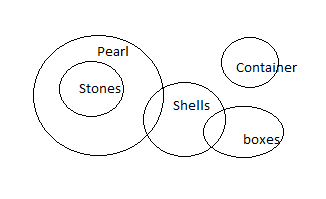I.Some stones are shells. This cannot be said with surety. As Stones and Shells may intersect or not.
II. No pearl is container.
III.No shell is container. The container set may or may not intersect with Shell set. No conclusion can be made.
IV.Some pearls are containers. Together II and IV form a universal set and either of them has to be true.

Only option C is correct.

The data can be intrepreted in Venn diagram form as: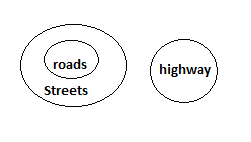Conclusions
I.No highway is a road. This is a correct conclusion.
II.All streets are road. This is a wrong conclusion.
Therefore, only conclusion I follows.

The data can be intrepreted in Venn diagram form as: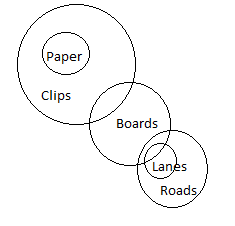I.Some roads are boards. This is correct conclusion.
II.Some lanes are clips. This is a wrong conclusion as apparent from the Venn diagram.
III.Some boards are papers. This is also a wrong conclusion.
IV.Some roads are clips. This is incorrect conclusion as apparent from Venn diagram.
Only I follows.

We use elimination in this syllogism. ie we draw an exception to contradict the conclusions. This diagram below contradicts I, II and IV. Conclusion II holds in various diagrams and can’t be contradicted. So, only conclusion II is implied. Since none of the options shows that, we choose (e) None of these.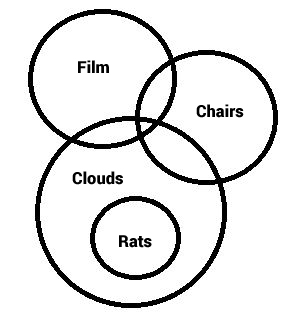The venn diagram for above statements is :

Conclusions:

I. Some buses are definitely not trucks = true
II. At least some trucks are cars = true

Thus, both conclusion I and II follow.

=> Ans – (D)

from the above statements we can draw the following venn

and hence we can say that either of the statements will be true

We use elimination to find an exception to the generality of the conclusions. Thus we prove they are not implied. The diagram below satisfies all the statements but contradicts the conclusions III and IV. Since we found an exception, the conclusions are not true in “every” case. Thus they are not implied.

We can draw many scenarios using Venn diagrams. Conclusions I and II hold in every case. So, they are implied.